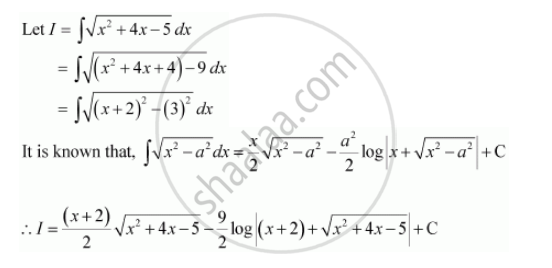Share

# Integrate the Functions Sqrt(X^2 + 4x - 5) - CBSE (Science) Class 12 - Mathematics

ConceptIntegrals of Some Particular Functions

#### Question

Integrate the functions sqrt(x^2 + 4x - 5)

#### SolutionIs there an error in this question or solution?

#### Video TutorialsVIEW ALL 

Solution Integrate the Functions Sqrt(X^2 + 4x - 5) Concept: Integrals of Some Particular Functions.
S